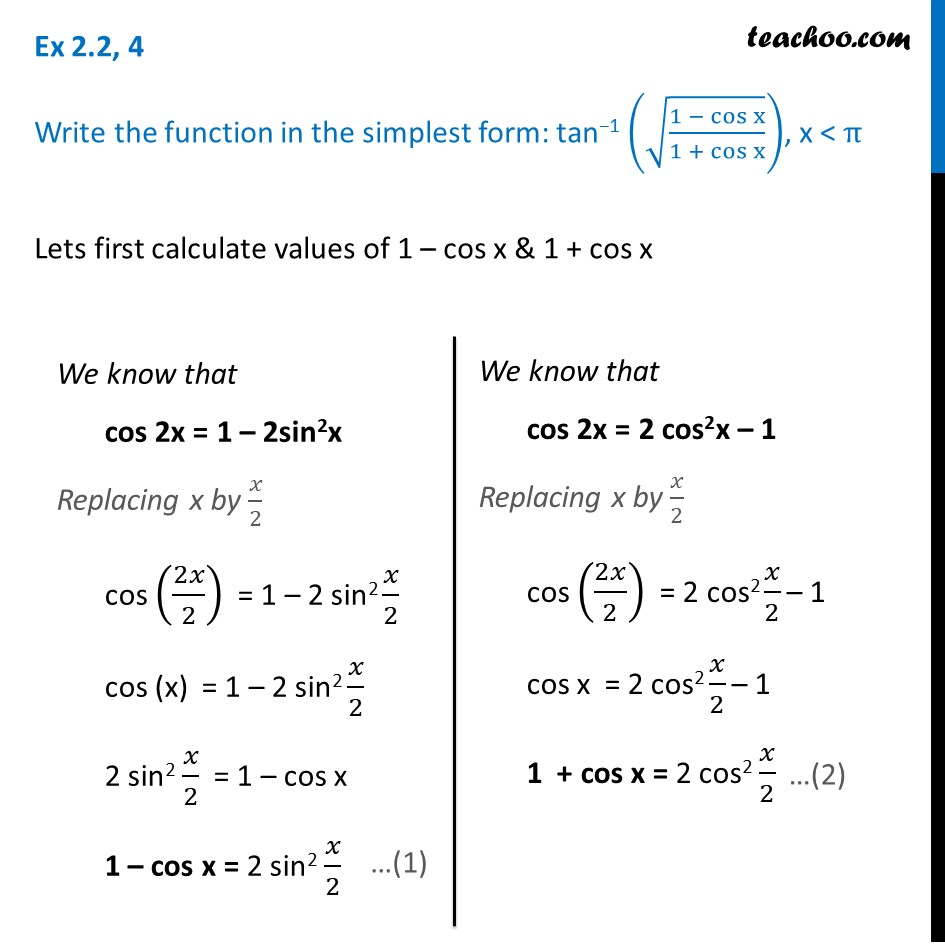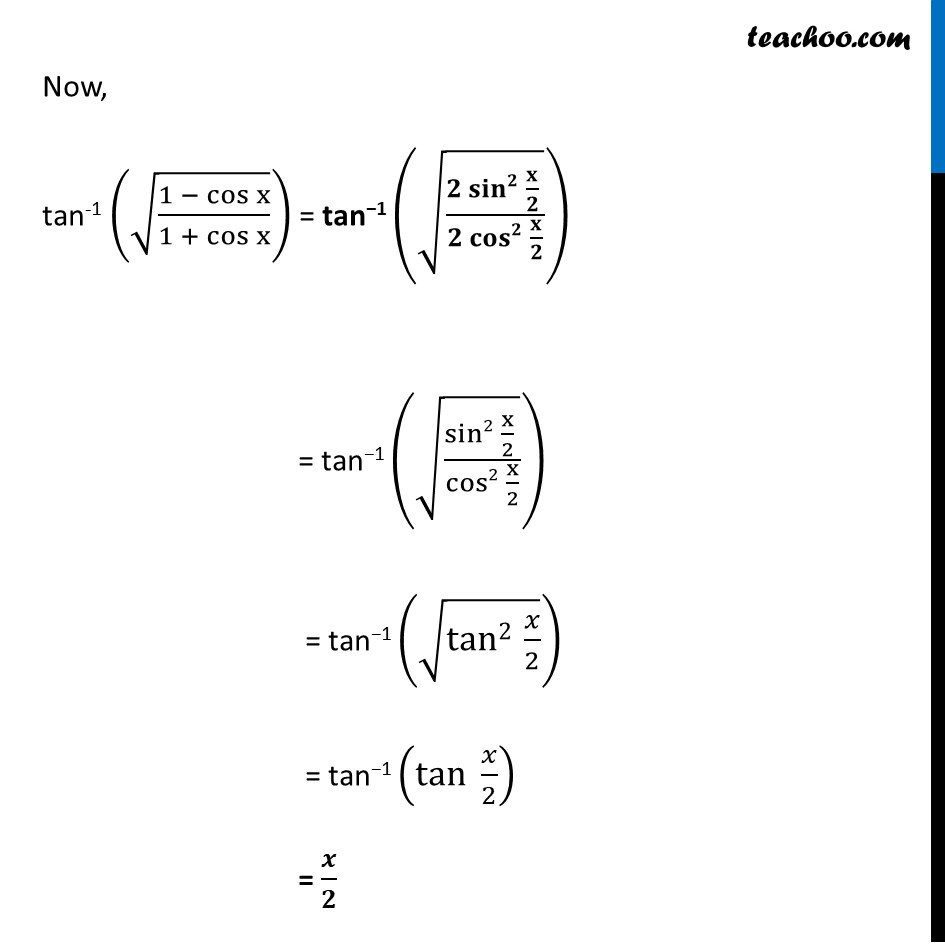Ex 2.2

Chapter 2 Class 12 Inverse Trigonometric Functions
Serial order wiseLearn in your speed, with individual attention - Teachoo Maths 1-on-1 Class

### Transcript

Ex 2.2, 4 Write the function in the simplest form: tan−1 (√((1 −〖 cos〗⁡x)/(1 +〖 cos〗⁡x ))), x < π Lets first calculate values of 1 – cos x & 1 + cos x We know that cos 2x = 1 – 2sin2x Replacing x by 𝑥/2 cos (2𝑥/2) = 1 – 2 sin2 𝑥/2 cos (x) = 1 – 2 sin2 𝑥/2 2 sin2 𝑥/2 = 1 – cos x 1 – cos x = 2 sin2 𝑥/2 We know that cos 2x = 2 cos2x – 1 Replacing x by 𝑥/2 cos (2𝑥/2) = 2 cos2 𝑥/2 – 1 cos x = 2 cos2 𝑥/2 – 1 1 + cos x = 2 cos2 𝑥/2 Now, tan-1 (√((1 −〖 cos〗⁡x)/(1 +〖 cos〗⁡x ))) = tan−1 (√((𝟐 𝐬𝐢𝐧𝟐 𝐱/𝟐 )/(𝟐 𝐜𝐨𝐬𝟐 𝐱/𝟐))) = tan−1 (√((sin2 x/2 )/(cos2 x/2))) = tan−1 (√(tan2⁡〖 𝑥/2〗 )) = tan−1 (tan⁡〖 𝑥/2〗 ) = 𝒙/𝟐